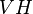# Normal equals potentially characteristic

This article gives a proof/explanation of the equivalence of multiple definitions for the term normal subgroup
View a complete list of pages giving proofs of equivalence of definitions

## Statement

The following are equivalent for a subgroup$H$ of a group$G$ :

1.$H$ is a normal subgroup of$G$.
2.$H$ is a potentially characteristic subgroup of$G$ in the following sense: there exists a group$K$ containing$G$ such that$H$ is a characteristic subgroup of$K$.

## Proof

### Proof of (1) implies (2) (hard direction)

Given: A group$G$, a normal subgroup$H$ of$G$.

To prove: There exists a group$K$ containing$G$ such that$H$ is characteristic in$K$.

Proof:

Step no. Assertion/construction Facts used Given data used Previous steps used Explanation
1 Let$S$ be a simple non-abelian group that is not isomorphic to any subgroup of$G$. Note that such a group exists. For instance, we can take the finitary alternating group on any set of cardinality strictly bigger than that of$G$.
2 Let$K$ be the restricted wreath product of$S$ and$G$, where$G$ acts via the regular action of$G/H$ and let$V$ be the restricted direct power$S^{G/H}$. In other words,$K$ is the semidirect product of the restricted direct power$V = S^{G/H}$ and$G$, acting via the regular group action of$G/H$. Step (1)
3 Any homomorphism from$V$ to$G$ is trivial. Steps (1), (2) By definition,$V$ is a restricted direct product of copies of$S$. Since$S$ is simple and not isomorphic to any subgroup of$G$, any homomorphism from$S$ to$G$ is trivial. Thus, any homomorphism from$V$ to$G$ is trivial.
4$V$ is characteristic in$K$. Steps (2), (3) Under any automorphism of$K$, the image of$V$ is a homomorphic image of$V$ in$K$. Its projection to$K/V \cong G$ is a homomorphic image of$V$ in$G$, which is trivial by step (3), so the image of$V$ in$K$ must be in$V$.
5 The centralizer of$V$ in$VH$ equals$H$. Steps (1), (2) By definition of the wreath product action,$H$ centralizes$V$. Since$S$ is centerless,$V$ is also centerless. Thus,$C_{VH}(V)$ contains$H$ but has trivial intersection with$V$, forcing$C_{VH} (V) = H$.
6 The centralizer of$V$ in$K$ equals$H$. Steps (2), (5) Step (5) already shows that$C_{VH}(V) = H$, so it suffices to show that$C_K(V) \le VH$. To see this, note that, by the construction in step (2), any element of$K$ outside$VH$ permutes the direct factors of$V$ as an element of$G$ outside$H$. This action is nontrivial, so the action is nontrivial, and hence elements outside$VH$ cannot centralize$V$. This forces$C_K(V) \le VH$, completing the proof.
7$H$ is characteristic in$K$. Fact (1) Steps (4), (6) Step-fact combination direct.
This proof uses a tabular format for presentation. Provide feedback on tabular proof formats in a survey (opens in new window/tab) | Learn more about tabular proof formats|View all pages on facts with proofs in tabular format

### Proof of (2) implies (1) (easy direction)

Given: A group$G$, a subgroup$H$ of$G$, a group$K$ containing$G$ such that$H$ is characteristic in$K$.

To prove:$H$ is normal in$G$.

Proof:

Step no. Assertion/construction Facts used Given data used Previous steps used Explanation
1$H$ is normal in$K$. Fact (2)$H$ is characteristic in$K$ -- Given-fact-combination direct.
2$H$ is normal in$G$. Fact (3)$H \le G \le K$ Step (1) Given-step-fact combination direct.